SIGNIFICANT FIGURES

For measured numbers, significant figures relate the certainty of the measurement. As the number of significant figures increases, the more certain the measurement. The means for obtaining the measurement also becomes more sophisticated as the number of significant figures increase.
Scientific notation is the most reliable way of expressing a number to a given number of significant figures. In scientific notation, the power of ten is insignificant. For instance, if one wishes to express the number 2000 to varying degrees of certainty:

2000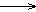2 x 103 is expressed to one significant figure

20002.0 x 103 is expressed to two significant figures

20002.00 x 103 is expressed to three significant figures

20002.000 x 103 is expressed to four significant figures

What do these numbers imply as to the certainty? Let's see what the number can be distinguished from:

The number 2000 to one significant figure lies between:

1 x 103 = 1000
2 x 103 = 2000
3 x 103 = 3000

It is a number that lies between 1000 and 3000 -- not very certain, is it.

The number 2000 to two significant figures lies between:

1.9 x 103 = 1900
2.0 x 103 = 2000
2.1 x 103 = 2100

It is a number that lies between 1900 and 2100 -- more certain than before.

The number 2000 to three significant figures lies between:

1.99 x 103 = 1990
2.00 x 103 = 2000
2.01 x 103 = 2010

It is a number that lies between 1990 and 2010 -- more certain, still.

The number 2000 to four significant figures lies between:

1.999 x 103
2.000 x 103
2.001 x 103

It is a number that lies between 1999 and 2001 -- even more certain.

The more significant figures in a measurement, the more sophisticated the means of measurement. You will see this in the laboratory. To measure out 200 mL of a solution using the marking stamped on the side of the beaker is quick and easy, but it is not as certain as a volumetric flask. When using a volumetric flask, care must be taken to bring the meniscus of the solution to a calibrated etch mark on the flask. Also, beakers are inexpensive pieces of glassware; volumetric flasks, because they are calibrated, are much higher in cost.

Being careless with significant figures may result in dire consequences. The following is a true story told to me by a Baltimore County middle school teacher concerning their mishap resulting from not considering the significance of significant figures:

The science teachers at a Baltimore County middle school wished to acquire a steel cube, one cubic centimeter in size to use as a visual aid to teach the metric system. The machine shop they contacted sent them a work order with instructions to draw the cube and specify its dimensions. On the work order, the science supervisor drew a cube and specified each side to be 1.000 cm. When the machine shop received this job request, they contacted the supervisor to double check that each side was to be one centimeter to four significant figures. The science supervisor, not thinking about the "logistics", verified four significant figures. When the finished cube arrived approximately one month later, it appeared to be a work of art. The sides were mirror smooth and the edges razor sharp. When they looked at the "bottom line", they were shocked to see the cost of the cube to be \$500! Thinking an error was made in billing, they contacted the machine shop to ask if the bill was really \$5.00, and not \$500. At this time, the machine shop verified that the cube was to be made to four significant figure specifications. It was explained to the school, that in order to make a cube of such a high degree of certainty, in addition to using an expensive alloy with a low coefficient of expansion, many man hours were needed to make the cube. The cube had to be ground down, and measured with calipers to within a certain tolerance. This process was repeated until three sides of the cube were successfully completed. So, "parts and labor" to prepare the cube cost \$500. The science budget for the school was wiped out for the entire year. This school now has a steel cube worth its weight in gold, because it is a very certain cubic centimeter in size.

When handling significant figures in calculations, two rules are applied:

Multiplication and division -- round the final result to the least number of significant figures of any one term, for example: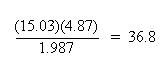The answer, 36.8, is rounded to three significant figures, because least number of significant figures was found in the term, 4.87. The other terms, 15.03 and 1.987, each had 4 significant figures.

Addition and subtraction -- round the final result to the least number of decimal places, regardless of the significant figures of any one term, for example: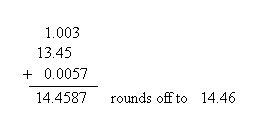The answer, 14.4587, was rounded to two decimal places, since the least number of decimal places found in the given terms was 2 (in the term, 13.45).

Suppose more than one mathematical operation is involved in the calculation? Such a calculation may be "deceptive" as to how many significant figures are actually involved. For instance: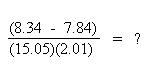The subtraction in the numerator must be performed first to establish the number of significant figures in the numerator. The subtraction results in: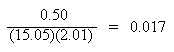Since the subtraction in the numerator resulted in a number to two significant figures (rounding to two decimal places), and the least number of significant figures in the resulting expression involving multiplication and division is now two significant figures, the final result must be rounded to two significant figures.

Created With HTML Assistant Pro - 05/11/2001 © Copyrght, 2001, L. Ladon. Permission is granted to use and duplicate these materials for non-profit educational use, under the following conditions: No changes or modifications will be made without written permission from the author. Copyright registration marks and author acknowledgement must be retained intact.